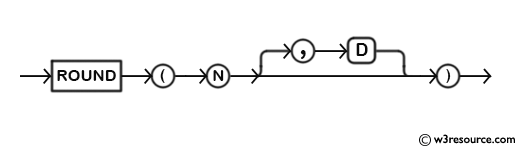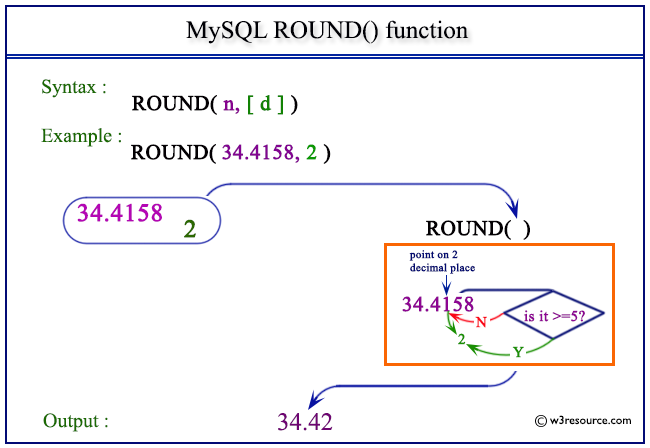# MySQL ROUND() function

## ROUND() function

MySQL ROUND() rounds a number specified as an argument up to a number specified as another argument.

This function is useful in -

• ROUND() allows you to control the precision of numeric values, ensuring that they have a specific number of decimal places.
• In data analysis and reporting, ROUND() is useful for aggregating and summarizing data.
• ROUND() is commonly used in financial applications for handling currency values.
• In cases where the result of a calculation might exceed the maximum precision of the data type, ROUND() can be used to prevent overflow errors and maintain data integrity.
• ROUND() can be used to facilitate numeric comparisons.
• When performing statistical calculations, such as calculating means or variances, ROUND() can be applied to the results to maintain consistent precision throughout the analysis.

Syntax:

```ROUND(N,[D]);
```

Argument:

Name Description
N A number which will be rounded upto D decimal places.
D A number indicating up to how many decimal places N will be rounded.

Note: The arguments can be zero(0) or negative. The default value of ‘D’ is 0 if not specified. if ‘D’ is 0, the round will happen from the left of the ‘D’ decimal point of the value ‘N’.

Syntax Diagram:MySQL Version: 8.0

Pictorial presentation of MySQL ROUND() functionExample of MySQL ROUND() function

Code:

``````SELECT ROUND(4.43);
```
```

Explanation:

The above MySQL statement will round the given number 4.43. No decimal places have been defined, so the default decimal value is 0.

Output:

```mysql> SELECT ROUND(4.43);
+-------------+
| ROUND(4.43) |
+-------------+
|           4 |
+-------------+
1 row in set (0.00 sec)
```

Example: ROUND() function with negative value

Code:

``````SELECT ROUND(-4.53);
```
```

Explanation:

The above MySQL statement will round the given number -4.53. No decimal places have been defined, so the default decimal value is 0.

Output:

```mysql> SELECT ROUND(-4.53);
+--------------+
| ROUND(-4.53) |
+--------------+
|           -5 |
+--------------+
1 row in set (0.00 sec)
```

Example: ROUND() function using decimal places

Code:

``````SELECT ROUND(-4.535,2);
```
```

Explanation:

The above MySQL statement will round the given number -4.535 up to 2 decimal places.

Output:

```mysql> SELECT ROUND(-4.535,2);
+-----------------+
| ROUND(-4.535,2) |
+-----------------+
|           -4.54 |
+-----------------+
1 row in set (0.00 sec)
```

Example: ROUND() function using negative decimal places

Code:

``````SELECT ROUND(34.4158,-1);
```
```

Explanation:

The above MySQL statement will round the given number 34.4158 from the left of decimal place up to 1 place.

Output:

```mysql> SELECT ROUND(34.4158,-1);
+-------------------+
| ROUND(34.4158,-1) |
+-------------------+
|                30 |
+-------------------+
1 row in set (0.00 sec)
```

All Mathematical Functions

Previous: RAND()
Next: SIGN()

﻿